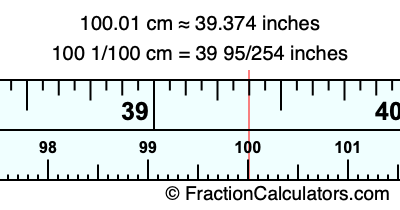100.01 cm in inchesHere is how to convert 100.01 cm to inches. We will give you the fractional answer, the decimal answer, and illustrate the answer on our tape measure.

One centimeter is equal to 50/127 inches, and 100.01 as a fraction is 100 1/100. Therefore, to get the fractional answer to 100.01 cm in inches, we multiply 100 1/100 by 50/127 and simplify if necessary.

cm × 50/127 = inches
100 1/100 × 50/127 = inches
100 1/100 × 50/127 = 39 95/254
100 1/100 cm = 39 95/254 inches
100.01 cm = 39 95/254 inches

As promised above, we also have the answer to 100.01 cm in inches in decimal form. Although the fractional answer above is exact, the decimal answer may be rounded if necessary:

39 95/254 ≈ 39.3740157480315
39 95/254 ≈ 39.374
100.01 cm ≈ 39.374 inches

Our image below shows 100.01 cm on a tape measure. The top row of the tape measure is inches, and the bottom row is centimeters.As you can see, we drew a red line where 100.01 cm and 39.374 inches meet on the measuring tape.

Centimeters to Inches Calculator
Here you can convert another length in centimeters to inches.

Convert  cm to inches.

What is 100.02 cm in inches?
Here is another inches to centimeters calculation we did for you.